# Analyzing the thermo-mechanical properties of a polymer material¶

Version: S-2021.06

In this tutorial you will learn how to estimate the thermo-mechanical properties of a polymer system, most notably the glass transition temperature, Young’s modulus and Poisson ratio. These properties are critical performance parameters in many applications. They can determine, for instance, the operating temperature or the maximum load of a given material. As these properties are determined by the atomic structure of the polymer system they can be estimated using molecular simulation.

The material used as an example will be a epoxy-amine thermoset material consisting of diglycidyl ether of bisphenol F (EPON-862) and diethylenetoluenediamine (DETDA). The tutorial Building an model of an epoxy thermoset material demonstrates the steps required to build a model of this material. This tutorial continues from that tutorial by demonstrating how the properties of the model can be simulated. The procedure outlined here is quite general, and can be applied to different types of polymeric and non-polymeric materials. Most notably, the same simulation procedure can be used on models of linear thermoplast polymers, such as poly(methyl metacrylate) and polyvinyl chloride, built using the Polymer Builder tools. It is not necessary that a polymer system be cross-linked for these methodology to give good results.

## Glass Transition Temperature¶

One important property of many polymer materials is the glass transition temperature. This is the temperature at which the material changes from a rigid, glassy state to a more flexible rubbery state. This transition from glassy to rubbery states in linear polymers usually corresponds to more torsional movement of the polymer chains as well as an increase in free volume. The glass transition temperature is typically estimated using a simulated annealing process, where the material is cooled during an NPT molecular dynamics simulation. Crossing the glass transition temperature also involves a change in the rate of thermal expansion, which can be identified by a change in the gradient of the density vs. temperature plot.

To calculate the glass transition temperature, an annealing simulation must first be performed. To set up this simulation, start by downloading the thermoset polymer model. Send this model to the Scripterby first opening it in the Builder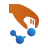and then using the iconto send the configuration to the Scripter. Alternatively you can save the HDF5 file in your project directory, open the file on the Lab Floor, and then drag the Bulk Configuration directly onto the Scripter. Start by adding a ForceField calculator. Open the calculator block and using the buttons on the right select New –> OPLS-AA to add an OPLS-AA forcefield. The next step is to equilibrate the system at an appropriate starting temperature. This can be achieved by adding a PolymerEquilibration block from Study Objects. The most important parameter to set here is the final temperature for equilibration. This temperature should be high enough above the glass transition temperature that it enables getting an estimate of the thermal expansion rate above the glass transition temperature. Open the PolymerEquilibration block and set the Final Temperature to 600K. The remaining default parameters are all appropriate. Once the system is properly equilibrated the annealing calculation can be performed. To do this, add a MolecularDynamics block from the Optimization group. Open this block and make the following changes:

• Select the NPT Martya Tobias Klein ensemble.
• Set the Steps to 1750000 and Log interval to 1000.
• In the Measurements section, add measurements for temperature, volume and density.
• Set the initial velocity to Configuration velocities.
• Set Reservoir temperature to 600K and Final temperature to 250K. Note that this sets a temperature sweep of 350K over 1.75ns at a rate of 0.2K/ps.

The remaining default parameters are suitable. The final molecular dynamics dialog is shown in the figure below.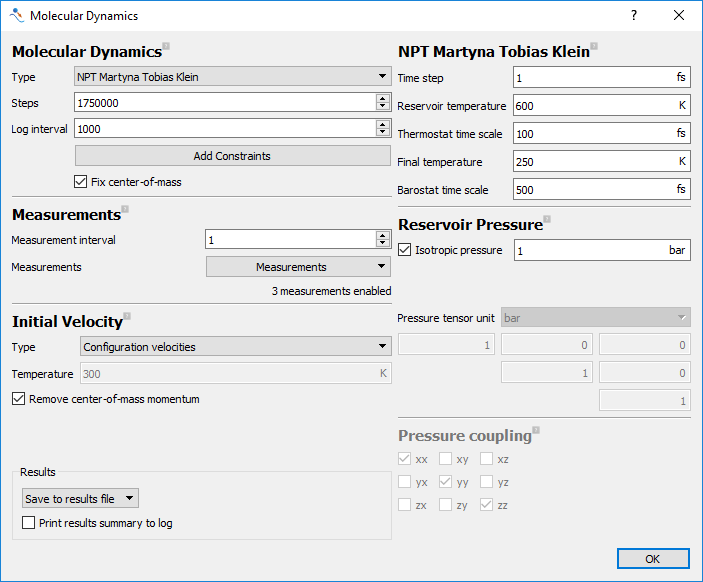Fig. 13 Settings for the annealing calculation to estimate Tg.

The script is now ready to be run. Send it to the Job Manager and run the script by using the Send To icon. This will produce the annealing trajectory that can be used to estimate the glass transition temperature. Note that as the script is performing over 3 million molecular dynamics steps, running it will take several hours.

Once the calculation is completed, open the trajectory from the Lab Floor using the Glass Transition Temperature Analyzer. This tool provides a plot of the density of the system with respect to temperature. It also estimates the glass transition temperature through fitting lines to the low and high temperature data, and then calculating the temperature at which the lines intersect. Click the Estimate fit limits button to automatically estimate the glass transition temperature. This adds limits indicating the high and low temperature fitting regions, as well as an estimate of the glass transition temperature. The limits can be manually adjusted to improve the quality of the fit. The glass transition temperature analyzer using the example calculation is shown in the figure below. This shows a glass transition temperature of 417K. This is in agreement with the range of experimental estimates of the glass transition temperature of this material .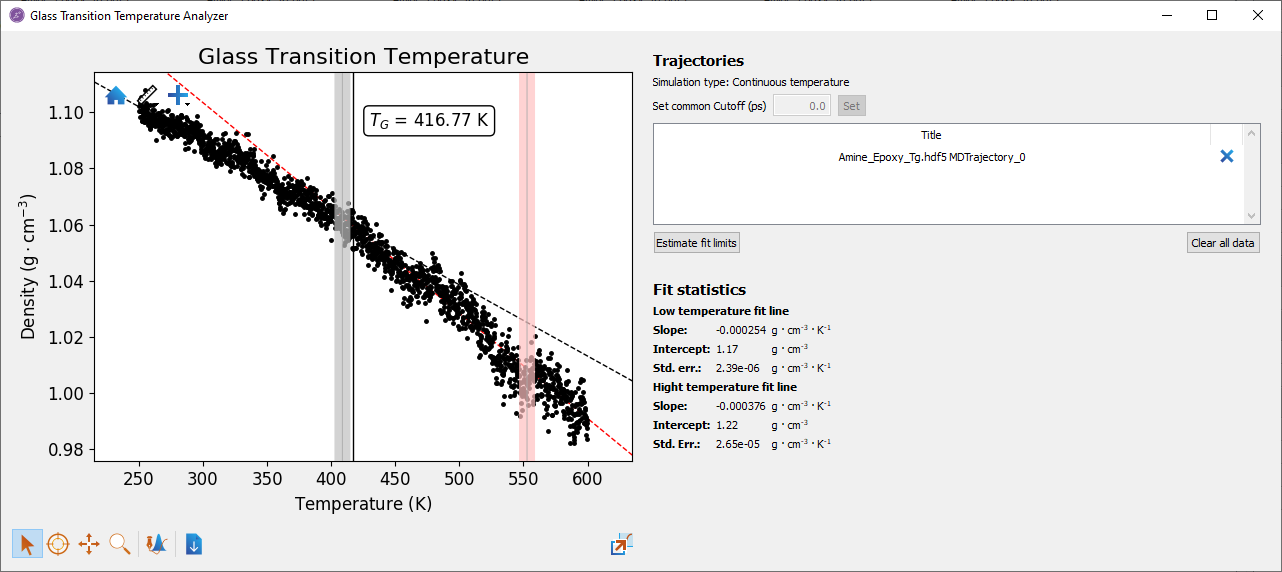Fig. 14 Glass transition temperature analyzer showing predicted Tg.

## Young’s Modulus and Poisson Ratio¶

Another important property of many epoxy systems is the Young’s Modulus. This is a measure of the amount of stress required to produce a given strain in the elastic deformation region. The Young’s modulus can be directly simulated using molecular dynamics. Here the strain is added by by progressively extending one of the simulation cell lengths. The resulting stress in this direction is then recorded. The gradient of the stress vs. strain curve then gives the Young’s modulus.

To carry out a simulation of the elastic properties of the material, a starting structure first must be selected. In the previous step a simulated annealing calculation was carried out, resulting in structures close to equilibrium at a range of temperatures. One option for selecting a starting structure is to take configurations from this annealing trajectory at the temperatures of interest. As these configurations are close to equilibrium, they may only need a relatively short equilibration calculation of around 100ps at the temperature of interest before starting the stress-strain simulation. This is possible because in the annealing calculation the starting configuration was first well-equilibrated at the starting temperature. As the Young’s modulus can change significantly, especially when crossing the glass transition temperature, this can be an efficient way to calculate the Young’s modulus at a range of temperatures. If the glass transition temperature has not been previously calculated, it is then still advisable to ensure that the starting configuration is well equilibrated at the temperature of interest using a reliable equilibration protocol. This is the approach that will be used in the following calculations, where the Young’s modulus of the example material will be estimated at room temperature.

To start setting up the calculation of Young’s modulus, open the final structure from the Crosslink Builder in the Scripter. As with the glass transition temperature, start by adding a Forcefield Calculator and then a PolymerEquilibration block from Study Objects. Open the PolymerEquilibration block and check the default parameters. As the system is being equilibrated to room temperature, all of the default parameters are appropriate. The required molecular dynamics calculations for simulating the stress-strain relationship can now be added. As the epoxy-main material is isotropic, the Young’s modulus should be the same in each dimension. The Young’s modulus can therefore be calculated in each direction and the average taken to provide a final value. Add 3 MolecularDynamics blocks from Optimization to the script. Each block will be used to simulate the Young’s modulus in a specific direction. Open the first MolecularDynamics block and set the following parameters.

• Set the Type to Stress Strain.
• In the Measurements section, add all of the diagonal (xx, yy and zz) strains.
• In the Initial Velocity section, set Type to Configuration velocities.
• In the Stress Strain MD section, select the xx as the Strain Direction. Also set the the final strain to 20%.

Note

In this simulation the cell is being strained by 20%. This is much larger than strictly required for a Young’s modulus calculation. Typically a total strain of around 2% is required is required for an estimate of the Young’s modulus. Here a larger strain is being used to illustrate the yield point on the stress-strain plot. As the calculated Young’s modulus can depend on the strain rate, it is generally better to use a lower strain rate.

Repeat the same steps for the remaining two molecular dynamics blocks, except in these set the Strain direction to yy and zz respectively. For setting the strain in the X direction, the molecular dynamics dialog should look like the one below.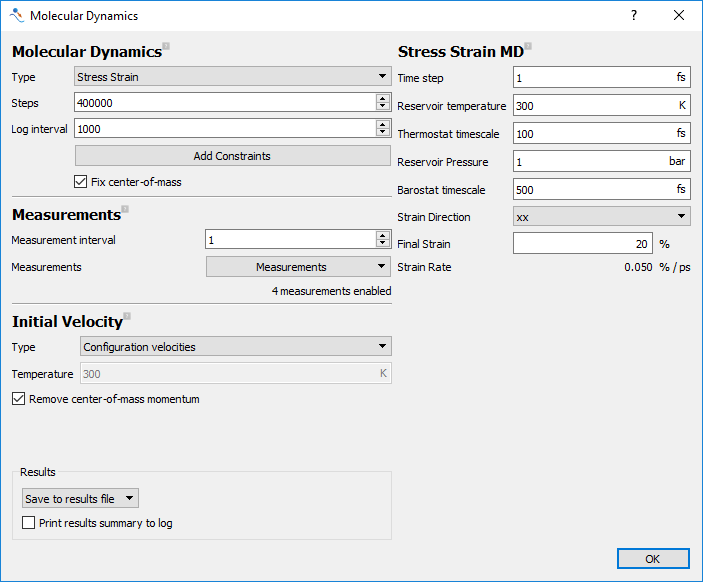Fig. 15 Settings for the annealing calculation to estimate Tg.

Before running the script, a few simple manual edits need to be performed. Open the script by sending it to the Editor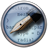using the Send To icon. By default calculations are chained together so that the resulting configuration from one calculation is used as the input for the next. In this case however, that would result in the material strained in one direction would be used as the input for straining in the next direction. The script needs to be changed so each stress-strain simulation starts from the same equilibrated structure. The links connecting each molecular dynamics calculation should be removed. These links occur in the line:

Here the starting bulk configuration is being over-written with the final bulk configuration from the molecular dynamics simulation. Delete this after each stress-strain molecular dynamics calculation. This will mean that the bulk configuration is not updated, resulting in the same bulk configuration being used as input for each dynamics simulation.

With these changes the script is now ready to run. Send it to the Job Manager and run the script by using the Send To icon. The final output of the calculation will be three molecular dynamics trajectories with the longitudinal stress and the three diagonal strains recorded in it. As before, since this script performs 2.8ns of molecular dynamics it can take several hours to run.

Once the calculation is finished, open the resulting HDF5 file on the Lab Floor. Open one of the MDTrajectories in the Movie Tool and observe the trajectory. You should see the material being extended along the longitudinal direction, while the material shrinks in the transverse directions. In the stress-strain simulation an NPT ensemble is used, allowing the transverse directions to respond according to the applied strain. To calculate the Young’s modulus a plot of the stress with respect to the strain should be created. In the Lab Floor, open the Data Plot tool. From the first trajectory drag the strain in the xx direction onto the X axis, and the xx stress only the Y axis. Click Create line to generate the plot.

In general, stress can fluctuate widely during an NPT molecular dynamics calculation compared to the stress resulting from the applied strain. This can obscure some of the important details of the stress-strain plot. To make it clearer, open the Plot Editor by clicking on the Edit icon at the bottom-left of the plot. This window shows the different elements in the plot and enables editing their properties. Select the curve and set the Opacity in the Plot Editor to 0.1. Right-click on the curve and select Add analysis ‣ Rolling average. This will help to average out some of the fluctuation in the stress. Select the rolling average, move the ranges to the edges of the plot and in the Plot Editor set Window to 1000. The rolling average should be linear at low strain, and then deviate from linearity at higher strain. To find the gradient at low strain right-click on the original plot and select Add analysis‣Polynomial fit. This adds a line of best fit onto the plot. Use the fitting ranges to move the line over the initial linear region of the stress-strain plot. The gradient and intercept of the line is shown in the Plot Editor with the Fit line selected. It is also shown by simply hovering the mouse over the line. Below is an example plot from the model system strained in the X direction.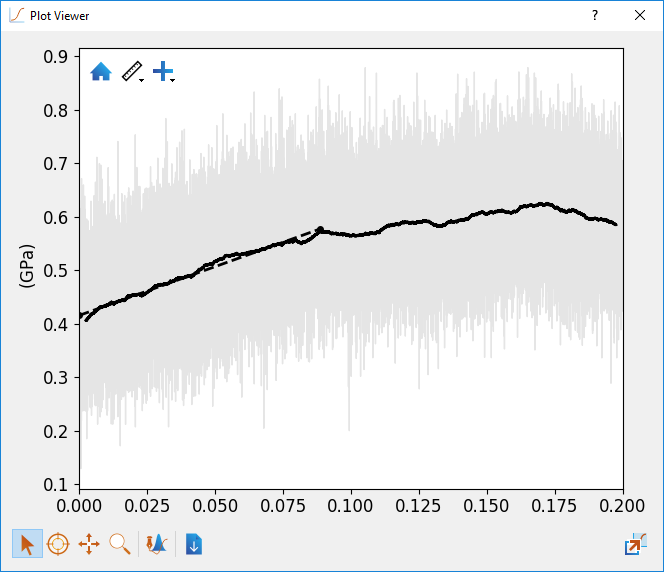Fig. 16 Plot of stress with respect to strain in the X direction in the epoxy-amine thermoset material.

A similar procedure can be used to estimate the Poisson ratio. This is the ratio of the strain in transverse direction to the applied strain. In each simulation the strain in each direction was saved in the MDTrajectoy. These can be plotted in the same way as the stress and strain to produce plots that can be fitted with lines of best fit. The gradient of these lines is then the Poisson ratio.

In the example calculation, averaging over the 3 strain directions and 6 Poisson ratios gives an average Young’s modulus of 2.0GPa and Poisson ratio of 0.35. The Young’s modulus in this case is lower than measured experimental values. This is likely due to the incomplete cross-linking reaction in the initial building of the epoxy-amine material. The starting configuration used in this tutorial was obtained from the Building an model of an epoxy thermoset material tutorial, which used a relatively low number of steps between cross-linking reactions. Increasing the number of NVT and NPT steps per cross-linking step increases the time between cross-linking events and also the distance that reactive atoms can move towards each other. In other simulations where the number of steps was set to 50000 for both NVT and NPT it was possible to link all reactant molecules into one large periodic structure. Increasing the degree of cross-linking results in a corresponding increase in the Young’s modulus. In calculations using a model built with more steps between cross-linking reactions, the same protocol for calculating the Young’s modulus resulted in values between 2.45GPa and 3.11GPa in the range of 90-95% completed cross-linking. This is in line with the experimentally measured Young’s modulus. The Poisson ratio however appears less sensitive to the degree of cross-linking. Here the calculated value of 0.35 is in agreement with the experimental value.

## Conclusions¶

In this tutorial the glass transition temperature, Young’s modulus and Poisson ratio of a thermoset polymer material were estimated using molecular dynamics simulation. Here these showed good agreement with the experimental glass transition temperature and Poisson ratio, while the Young’s modulus was somewhat less than the experimental value. This was due to the incomplete cross-linking in the original configuration due to the short time between cross-linking steps. Overall this tutorial shows how reliable models of complex thermoset materials can be easily created and their properties accurately simulated in QuantumATK. These methods can be successfully applied across a range of materials, including thermoset and thermoplast polymers.

References

  L. J. Abbott, K. E. Hart, C. M. Colina: Polymatic: a generalized simulated polymerization algorithm for amorphous polymers Theor. Chem. Acc. 132, 1334 (2013)
  (1, 2) K. Tao, S. Yang, J. C. Grunlan, Y. S. Kim, B. Dang, Y. Deng, R. L. Thomas, B. L. Wilson, X. Wei: Effects of carbon nanotube fillers on the curing processes of epoxy resin-based composites J. Appl. Polym. Sci. 102, 5248 (2006)
  (1, 2) L. Sun, G. L. Warren, J. Y. O’Reilly, W. N. Everett, S. M. Lee, D. Davis, D. Lagoudas, H. J. Sue: Mechanical properties of surface-functionalized SWCNT/epoxy composites Carbon 46, 320 (2008)
  J. L. Tack: Thermodynamic and mechanical properties of EPON 862 with curing agent DETDA by molecular simulation Master’s thesis, Texas A&M University (2006)